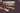# Dealing with randomness in tests

You can watch me go over the examples here:

Let's say we have a function that makes our user feel welcomed by picking randomly one of the available welcoming messages:

We would like to write a test for it, but it randomly fails - because the result of the function is also random. We have a few options, the first one would be to use a different sample function in a test while defaulting it to the one that introduces randomness:

``````// note the new optional argument
const welcomeUser = (userName, sample = _.sample) => {
const pickedMessage = sample(welcomeMessages);
};

// in test:
const sample = (arrayToGetSampleFrom) => arrayToGetSampleFrom;
expect(welcomeUser("Lukasz", sample)).toEqual("Hello there, Lukasz");``````

This is nice, and I would definitely advise applying this technique when needed, especially if you are doing a Unit Test.

If you are thinking about integration tests, or maybe even e2e tests, it won't be trivial to inject a dependency in a similar manner.

Usually, your app might have very few functions that use randomness (the frequent case is an "id generator" - defined once, but used frequently), so it might be helpful to just make them random in production, but non-random in tests, like so:

``````// sample.ts
import _ from "lodash";

export const sample = <T>(arrayToGetSampleFrom: T[]): T =>
process.env.TEST_ENV
? arrayToGetSampleFrom
: _.sample(arrayToGetSampleFrom);

// welcomeUser.ts
import {sample} from "./sample"

const welcomeMessages = ["Hello there", "Hi", "Goedemorgen"];
const welcomeUser = (userName) => {
const pickedMessage = sample(welcomeMessages);
};

``````

Note: you can use the same patterns for the dreaded dates :)

``````const getCurrentDate = () =>
process.env.TEST_ENV ? new Date(1616667648490) : new Date()``````

As for a bonus, let's take a look at the ultimate problem in randomness - random number generator.

I will use a real-life example since it's sufficiently small to be useful without introducing unnecessary details. We needed to have a function that would generate a number between 0 and 10. We used that to introduce a randomized delay for processing data (let's say we got a batch with hundreds of files every 30 minutes, so we would push them for processing in a queue with a randomized delay, so our system would not have to worry about huge spikes). The function was simple enough:

``````const getRandomNumberBetweenZeroAndTen = (): number => {
const minVal = 1
const maxVal = 10
return Math.floor(Math.random() * (maxVal - minVal)) + minVal
}

``````

And the test even simpler:

``````expect(getRandomNumberBetweenZeroAndTen()).not.toEqual(getRandomNumberBetweenZeroAndTen())
expect(getRandomNumberBetweenZeroAndTen() >= 0).toEqual(true)
expect(getRandomNumberBetweenZeroAndTen() <= 10).toEqual(true)``````

Our developer went to sleep smiling about his well-done job and smart algorithm.

The problem started very soon because his test would randomly fail with:

``````expect(received).not.toEqual(expected) // deep equality

Expected: not 3``````

As you probably guessed by now, there is a 1 in 10 chance that the two random executions of this function will result in the same number (not necessarily 3..). This is not really acceptable - and if you had 10 tests with a random chance of 10% failure then virtually all your builds will start failing, which will bring the development efforts to a halt.

So the solution is similar to our first example. We have to realize that the part we are testing is not the built-in NodeJS number generator - let's leave the NodeJS folks to take care of that. We want to verify our logic. To do that - let's take the random number as an optional argument.

``````const getRandomNumberBetweenZeroAndTen = (
randomNumber = Math.random()
): number => {
const minVal = 1
const maxVal = 10
return Math.floor(randomNumber * (maxVal - minVal)) + minVal
}
``````

And now we can test it nicely:

``````test(`returns a number between 1 and 10
proportionally based on the passed randomNumber that is between 0 and 1`, () => {
expect(getRandomNumberBetweenZeroAndTen(1)).toEqual(10)
expect(getRandomNumberBetweenZeroAndTen(0)).toEqual(1)
expect(getRandomNumberBetweenZeroAndTen(0.5)).toEqual(5)
expect(getRandomNumberBetweenZeroAndTen(0.15)).toEqual(2)
})``````

As a side-note and taking another step back to our initial example, in many cases, it would be a better idea to just use lodash random and possibly wrap it as well

``````export const random = (lower, upper) =>
process.env.TEST_ENV ? lower : _.random(lower, upper);
``````

For example - If you are dealing with a tiny lambda function without any external dependencies (outside the provided by AWS aws-sdk), it might be easier/faster to write some of those helpers yourself and not worry about external dependencies. I'd argue this is rarely the case, and it's almost always better to use the standard node.js ecosystem tooling. Nonetheless, you might end up in situations where you won't be able to escape from Math.random function, and I hope the patterns from this article will help you to deal with them.

Let me know if you have any questions or thoughts in the comments below.

We at Xolvio specialize in helping our clients get more for less. We can get you to the holy grail of continuous deployment where every commit can go to production — and yes, even for large enterprises.

Feel free to schedule a call or send us a message below to see how we can help.

or

Book a call
+